# Online Plane Geometry: Pascal's Mystic Hexagram Theorem Proof, Collinear Points. Level: High School, College, Mathematics Education

Let A, B, C, D, E, F be points on a circle and makes a hexagon out of them in an arbitrary order. Then the three points L, M, N at which pairs of opposite sides meet, lie on a straight line. This line is called the Pascal line of the hexagon ABCDEF.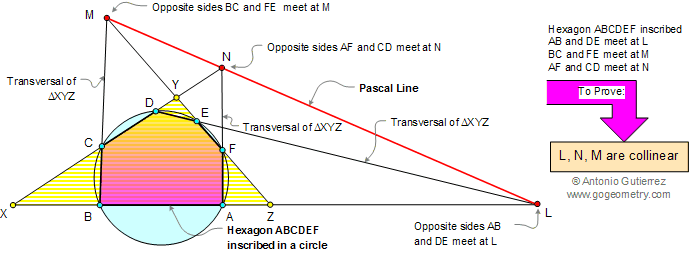# Proof:

1. Applying Menelaus’ Theorem respectively to transversal BCM, AFN, and DEL of triangle XYZ: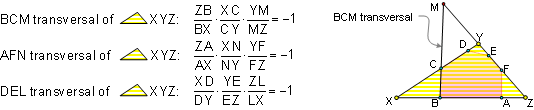2. Multiplying the three identities, we get: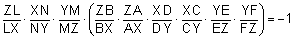3. Using the intersecting secant theorem, we get: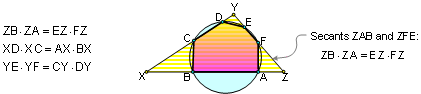4. By substituting (3) into (2), we get: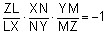Thus, by the converse of Menelaus' Theorem, points M, N, and L lie on a transversal of triangle XYZ, therefore must be collinear. Q.E.D.

Blaise Pascal (1623-1662) French mathematician, philosopher and inventor, discovered his famous theorem at the age of 16, in 1640, and produced a treatise on conic sections entitled Essai pour les coniques.